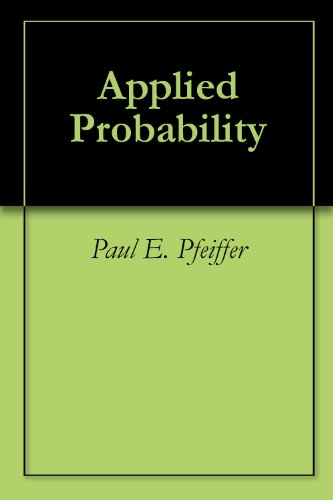Processing ......FreeComputerBooks.com Free Computer, Mathematics, Technical Books and Lecture Notes, etc.

Applied Probability
How many flights will arrive to a particular airport? Click here to find out.
• Title Applied Probability
• Author(s) Paul E Pfeiffer
• Publisher: Connexions 2012
• Hardcover/Paperback N/A
• eBook PDF (634 page, 6.2 MB)
• Language: English
• ISBN-10/ASIN: B005JZZMXQ
• ISBN-13: N/ABook Description

This is a 'first course' in the sense that it presumes no previous course in probability. The units are numbered as they appear in the text, although of course they may be used in any desired order. For those who wish to use the order of the text, an outline is provided, with indication of which modules contain the material.

The mathematical prerequisites are ordinary calculus and the elements of matrix algebra. A few standard series and integrals are used, and double integrals are evaluated as iterated integrals. The reader who can evaluate simple integrals can learn quickly from the examples how to deal with the iterated integrals used in the theory of expectation and conditional expectation.

In addition to an introduction to the essential features of basic probability in terms of a precise mathematical model, the work describes and employs user defined MATLAB procedures and functions (which we refer to as m-programs, or simply programs) to solve many important problems in basic probability. This should make the work useful as a stand alone exposition as well as a supplement to any of several current textbooks

Most of the programs developed here were written in earlier versions of MATLAB, but have been revised slightly to make them quite compatible with MATLAB 7. In a few cases, alternate implementations are available in the Statistics Toolbox, but are implemented here directly from the basic MATLAB program, so that students need only that program (and the symbolic mathematics toolbox, if they desire its aid in evaluating integrals).All CategoriesRecent BooksIT Research LibraryMiscellaneous BooksComputer LanguagesComputer ScienceData Science/DatabasesElectrical EngineeringJava and Java EE (J2EE)Linux and UnixMathematicsMicrosoft and .NETMobile ComputingNetworking and CommunicationsSoftware EngineeringSpecial TopicsWeb Programming